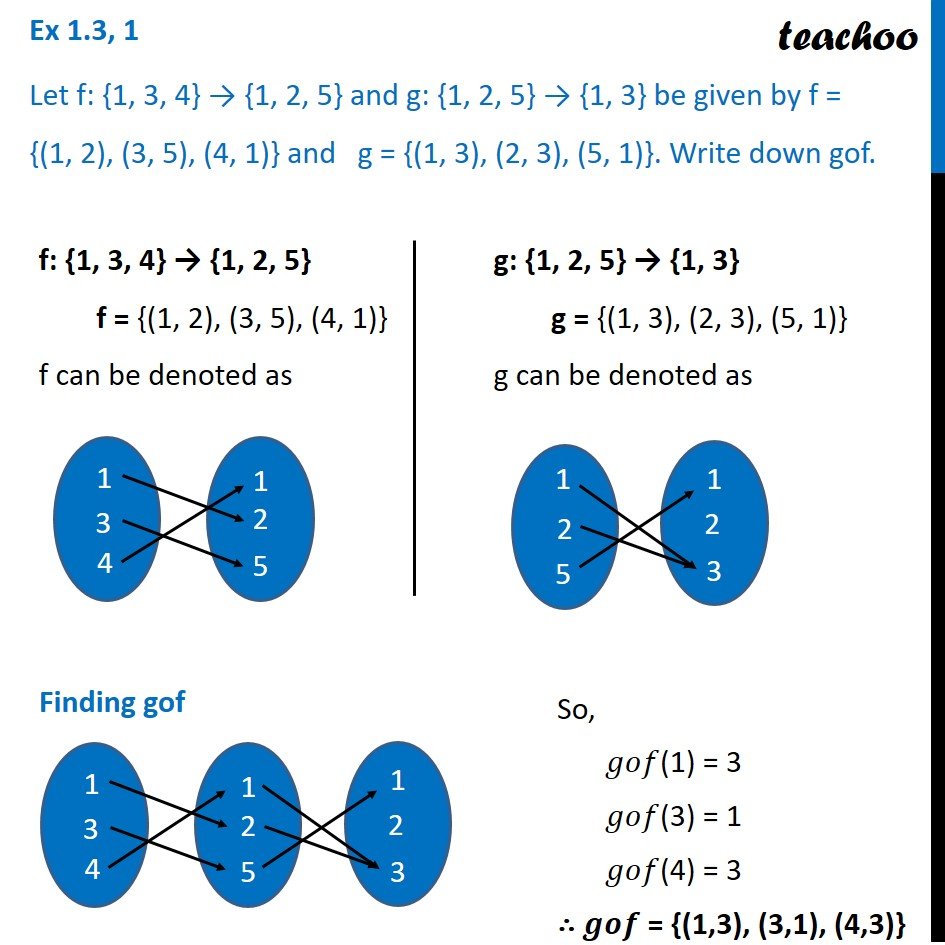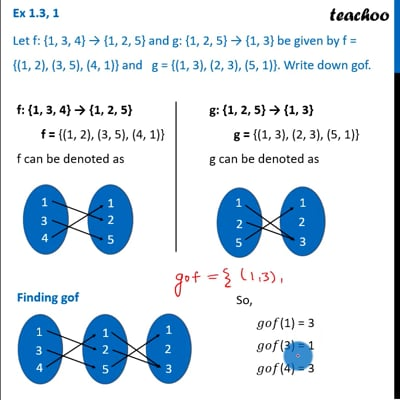Composite functions

Chapter 1 Class 12 Relation and Functions
Concept wiseThis video is only available for Teachoo black usersThis video is only available for Teachoo black users

Learn in your speed, with individual attention - Teachoo Maths 1-on-1 Class

### Transcript

Ex 1.3, 1 Let f: {1, 3, 4} → {1, 2, 5} and g: {1, 2, 5} → {1, 3} be given by f = {(1, 2), (3, 5), (4, 1)} and g = {(1, 3), (2, 3), (5, 1)}. Write down gof. f: {1, 3, 4} → {1, 2, 5} f = {(1, 2), (3, 5), (4, 1)} f can be denoted as g: {1, 2, 5} → {1, 3} g = {(1, 3), (2, 3), (5, 1)} g can be denoted as Finding gof So, 𝑔𝑜𝑓(1) = 3 𝑔𝑜𝑓(3) = 1 𝑔𝑜𝑓(4) = 3 ∴ 𝒈𝒐𝒇 = {(1,3), (3,1), (4,3)}